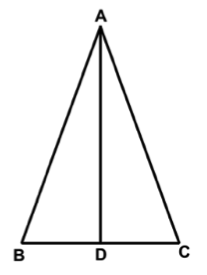### Prove: Triangle ABC is IsoscelesBD is congruent to DC
AD is the perpendicular bisector of BC
CPCTC
Reflexive
SAS (Side-Angle-Side)
AD is congruent to AD
All right angles are equal
angle ADB is congruent to angle ADC
AB is congruent to AC
Perpendicular lines form right angles
Given
angle ADB and angle ADC are right angles
Triangle ABD is congruent to Triangle ACD
Triangle ABC is Isosceles
Definition
Definition